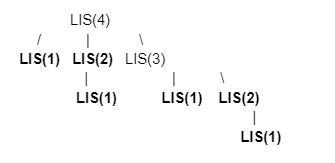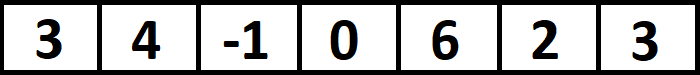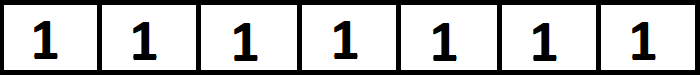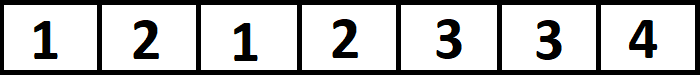# Longest Increasing Subsequence | Part 1

## Introduction

In this article, we will talk about the longest increasing subsequence problem. It is a trivial problem with solutions ranging from exponential complexity to logarithmic complexity.

Problems like longest increasing subsequence are asked in many coding competitions and coding interviews, directly or indirectly. Other than this, the longest increasing subsequence is also used in various places like:

• Git- for their version control system.
• MUMmer system- for aligning entire genomes.

I hope many of you readers are now excited to learn about the Longest Increasing Subsequence. So without any further ado, let’s start our journey.

## Problem Statement

For a given array with N elements, you need to find the length of the longest subsequence from the array such that all the elements of the subsequence are sorted in strictly increasing order.

A strictly Increasing Sequence is when each term in the sequence is larger than the preceding term.

Sample Input :

6

5 4 11 1 16 8

Sample Output :

3

Explanation:

Length of longest subsequence is 3 i.e. [5, 11, 16] or [4, 11, 16].

The increasing subsequence can start from any point and end at any point. It is also not certain that the first increasing subsequence which we have found is the longest. Thus we have to compute all the possible increasing subsequences and then find the longest among them.

For the ease of the readers this article is a two-part article. This is the first part of the article, which contains the iterative solution and a semi-optimized solution obtained by using DP. The second part of the article can be found here. Reading both articles will help you build up an efficient solution on your own in no time.

It is recommended to first practice the Longest Increasing Subsequence on CodeStudio before proceeding to the solution.

Without further ado, let’s get started.

## Approach 1 - Recursion

Consider the array A as {5,4,11,1,15,8}.

Consider a variable prev and a variable idx. For every element in A, we have two choices: either it is part of the LIS, or it is not.

Now is it always like it will have an option of being a part of LIS? The answer is NO because it can only be part of the LIS if and only if the previous element included in LIS is smaller than A[idx].

In our code, we have a function called LIS which will return the length of the longest increasing subsequence and will take three parameters as follows:

• idx: To store the index of the current element to be included or excluded.
• prev: To store the previously included element in LIS. Initially, we will pass it as the minimum value of an integer.
• Array A: The input array

OUTPUT

### Complexity Analysis

#### Time Complexity

In the code mentioned above, for every idx we have two possible options, either include or exclude the element in our LIS. Thus time complexity is:

T(n) = O(2n),

where n is the size of the input array.

#### Space Complexity

In the code mentioned above, we use recursion to find the LIS of the given input array. Thus,

Space Complexity = O(n),

where n is the space occupied by the recursion stack.

In the worst case, we have n functions in the recursion stack occupying O(n) memory.

The recursion tree obtained by this recursive approach is:We can see that the highlighted functions are being called multiple times. These redundant calculations drastically affect our time complexity. So, to avoid these redundant computations, we use the concept of Dynamic Programming. To know how to apply dynamic programming to this problem, let’s proceed to our following approach.

## Approach 2 - Dynamic Programming

We will have a 1D dp vector of the same size as the input array. The dp vector will store the length of the LIS, such that if the current number is the part of LIS. For this, we will find all the smaller numbers on the left of i and add 1 to the length obtained till that smaller number.

### Algorithm:

• Create a dp vector equal to the size of the input array and initialize all the elements as 1.
• Create a variable max to store the maximum length of LIS and initialize it as negative infinity.
• For every index idx in array A.
• For all indices j less than idx
• If A[j] < A[idx], then dp[idx] = Math.max(dp[idx], dp[j] + 1);
• Update max as max = (max, dp[idx]);

### Explanation:

Let us consider the input sequence {3, 4, -1, 0, 6, 2, 3}.

Thus the input array becomes:Since each element is an increasing subsequence in itself, we initialize all the elements of the dp array to 1. Thus, the dp array on initialization will look like this:On execution of the code, each position in the dp array denotes the length of the increasing subsequence from dp up to dp[i]. Thus after the code execution, the dp array changes to-To explain furthermore, we have:

• At dp, we have the length of the increasing subsequence, which starts at dp and ends at dp, and the elements are less than 3. Since there is only one element that fulfills this condition, we keep dp unchanged.
• At dp, we have the length of the increasing subsequence, which starts at dp and ends at dp, and the elements are less than 4. There are two elements {3,4} that fulfill this condition. Thus dp is updated to 2.
• At dp, we have the length of the increasing subsequence, which starts at dp and ends at dp, and the elements are less than -1. Again, only one element satisfies this condition, due to which the value remains unchanged.

Once we have populated the dp vector, we iterate through it to find the maximum element and print it.

Let’s see the C++ implementation of the above approach to get a better understanding.

OUTPUT

### Complexity Analysis

#### Time Complexity

In the above solution, we iterate for every index in vector dp for all the indexes less than the idx. Thus time complexity is,

T(n) = O(n2),

where n is the size of the input array.

#### Space Complexity

In the above solution, we maintain a 1D dp vector to store the size of the LIS. Thus,

Space Complexity = O(n),

where n is the size of the dp vector.

Continuous reading can be boring, so we summarized the whole article into a video tutorial for your convenience in understanding the logic of this problem.

1. What is the difference between substring vs subsequence?
A Substring takes out characters from a string placed between two specified indices in a continuous order. On the other hand, subsequence can be derived from another sequence by deleting some or none of the elements in between but always maintaining the relative order of elements in the original sequence.

2. Is there any other technique to solve the longest increasing subsequence problem?
Yes, we can use the binary search + dp technique to solve the longest increasing subsequence problem. Want to learn more about it, read longest increasing subsequence|part-2 here.

3. What are some other problems including subsequences?
Try the problems- Longest Common SubsequenceLongest Consecutive Subsequence on CodeStudio.

## Key Takeaways

To summarize this article, we had an in-depth understanding of the longest increasing subsequence problem. We first briefly saw the problem statement, followed by a vivid explanation of the recursive solution as well as an optimized solution using dynamic programming. The codes are made reader-friendly so that first-time learners can easily understand the problem and implement it on their own.

If you have reached this far, don’t forget to read longest increasing subsequence|part-2 here.

Want to solve coding problems asked in various IT companies? Practice such questions on our CodeStudio - The best platform to prepare for coding interviews